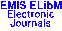## Publications de l'Institut Mathématique (Beograd)Vol. 50(64) Preimenovati datoteke, proveriti paginaciju!!!, 1991 · Contents

 Journal Home All Journals ELibM Home EMIS Home Milan Tasi{\cj}: The neg.-propositional calculus {\Z}arko Mijajlovi{\cj}: Definable ultrapowers and the omitting types theorem Dragos M. Cvetkovi{\cj}: Some comments on the eigenspaces of graphs Aleksandar Torga{\sh}ev: A property of canonical graphs Miroslav Petrovi{\cj}: Some results on graphs with at most two positive eigenvalues K. Ramachandra and A. Sankaranarayanan: Note on a paper by H.\,L. Montgomery Omega theorems for the Riemann zeta-function'' Barry J. Powell and Tibor {\v S}al{á}t: Convergence of subseries of the harmonic series and asymptotic densities of sets of positive integers Stojan Bogdanovi{\cj} and Miroslav {\CJ}iri{\cj}: Tight semigroups Mara Alagi{\cj}: Relations with $I$-structure in categories with pullbacks Dusan R. Georgijevi{\cj}: Radial $N$-th derivatives of bounded analytic operator functions M. Mateljevi{\cj} and M. Pavlovi{\cj}: Some inequalities of isoperimetric type concerning analytic and subharmonic functions Miroslav Pavlovi{\cj}: On isomorphisms of $L^1$ spaces of analytic functions onto $l^1$ Darko Milinkovi{\cj}: Trace formula for nuclear perturbations of discrete nonselfadjoint operators Milutin Dostani{\cj}: The regularized trace for nuclear perturbations of discrete operators Ljuban Dedi{\cj}: The dual Steiner formula for convex compacta Domingo Chinea, Manuel de León, and Juan C. Marrero: Symplectic and cosymplectic foliations on cosymplectic manifolds Publication date for this issue: 2 Nov 2001. This page was last modified: 16 Nov 2001. © 2001 Mathematical Institute of the Serbian Academy of Science and Arts © 2001 ELibM for the EMIS Electronic Edition